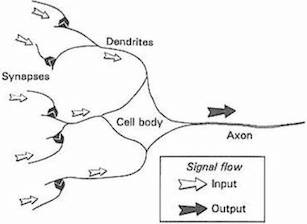“It were much to be desired, that when mathematical processes pass through the human brain instead of through the medium of inanimate mechanism, it were equally a necessity of things that the reasonings connected with operations should hold the same just place as a clear and well-defined branch of the subject of analysis, a fundamental but yet independent ingredient in the science, which they must do in studying the engine.” Sketch of the Analytical Engine (1843), Ada Lovelace

# 神经网络

translated by Tong Li & weakish

## 神经网络的诞生和再生Simplified neuron body within a networkSource: Gurney, 1997. An Introduction to Neural Networks

Frank Rosenblatt 在 20 世纪 50 年代后期发明了感知器（Perceptron），也就是我们在上一章看到的线性分类器，这对于神经网络是很大的进步。被美国海军公开资助的 Mark 1 感知器是用一串光电管、电位器、电机来实现图像识别功能。它能高效地执行复杂的电路操作，以至于纽约时报在 1958 年预测这个机器不久就能“走路，说话，观察，书写，自我复制和意识到自身的存在”。

• 它们的结构异常简单而符合直觉。

• 在和本书最为相关的若干机器学习任务中，深度神经网络是目前最先进的技术。

• 近来大多数机器学习的创意应用都是用神经网络来制作的。

## 从线性分类器到神经元

$\begin{eqnarray} f(x_1, x_2) = b + w_1 x_1 + w_2 x_2 \end{eqnarray}$

$\begin{eqnarray} f(X) = b + \sum_i w_i x_i \end{eqnarray}$

$\begin{eqnarray} \mbox{classification} & = & \left\{ \begin{array}{ll} 1 & \mbox{if } f(X) \gt 0 \\ 0 & \mbox{if } f(X) \leq 0 \end{array} \right. \tag{1}\end{eqnarray}$

## 激活函数

$\sigma(x) = \frac{1}{1 + e^{-x}}$

$e$代表指数常数，约等于2.71828。使用 sigmoid 作为激活函数的神经元叫做 sigmoid 神经元。我们首先令变量 $z$ 等于初始输入值的加权和，然后把它放入 sigmoid 函数中进行运算。

$z = b + \sum_i w_i x_i \\ \sigma(z) = \frac{1}{1 + e^{-z}}$

sigmoid 函数是连续可微的，而它的导数，很简单地，是 $\sigma^\prime(z) = \sigma(z) (1-\sigma(z))$。这很重要，因为我们需要用微积分来训练神经网络，但现在还不用担心这些。

$z = b + w_1 x_1 + w_2 x_2 + w_3 x_3 \\ y = \sigma(z)$